Olympiad Test : Mirror Images - 2

# Olympiad Test : Mirror Images - 2

Test Description

## 10 Questions MCQ Test Maths Olympiad Class 6 | Olympiad Test : Mirror Images - 2

Olympiad Test : Mirror Images - 2 for Class 6 2022 is part of Maths Olympiad Class 6 preparation. The Olympiad Test : Mirror Images - 2 questions and answers have been prepared according to the Class 6 exam syllabus.The Olympiad Test : Mirror Images - 2 MCQs are made for Class 6 2022 Exam. Find important definitions, questions, notes, meanings, examples, exercises, MCQs and online tests for Olympiad Test : Mirror Images - 2 below.
Solutions of Olympiad Test : Mirror Images - 2 questions in English are available as part of our Maths Olympiad Class 6 for Class 6 & Olympiad Test : Mirror Images - 2 solutions in Hindi for Maths Olympiad Class 6 course. Download more important topics, notes, lectures and mock test series for Class 6 Exam by signing up for free. Attempt Olympiad Test : Mirror Images - 2 | 10 questions in 40 minutes | Mock test for Class 6 preparation | Free important questions MCQ to study Maths Olympiad Class 6 for Class 6 Exam | Download free PDF with solutions
 1 Crore+ students have signed up on EduRev. Have you?
Olympiad Test : Mirror Images - 2 - Question 1

### In each of the following questions, choose the correct mirror image of the figure (X) from amongst the four alternatives (a), (b), (c) and (d) given along with Fig. (X).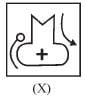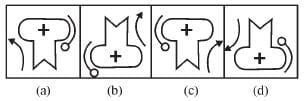Olympiad Test : Mirror Images - 2 - Question 2

### In each of the following questions, choose the correct mirror image of the figure (X) from amongst the four alternatives (a), (b), (c) and (d) given along with Fig. (X).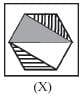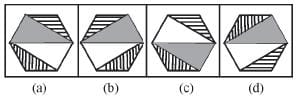Olympiad Test : Mirror Images - 2 - Question 3

### In each of the following questions, choose the correct mirror image of the figure (X) from amongst the four alternatives (a), (b), (c) and (d) given along with Fig. (X).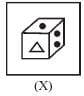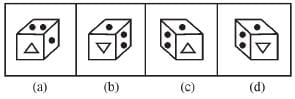Olympiad Test : Mirror Images - 2 - Question 4

In each of the following questions, choose the correct mirror image of the figure (X) from amongst the four alternatives (a), (b), (c) and (d) given along with Fig. (X).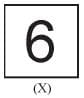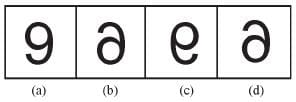Olympiad Test : Mirror Images - 2 - Question 5

In each of the following questions, choose the correct mirror image of the figure (X) from amongst the four alternatives (a), (b), (c) and (d) given along with Fig. (X).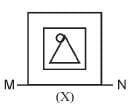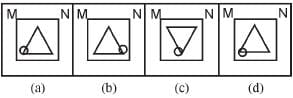Olympiad Test : Mirror Images - 2 - Question 6

In each of the following questions, choose the correct mirror image of the figure (X) from amongst the four alternatives (a), (b), (c) and (d) given along with Fig. (X).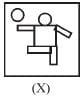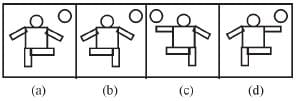Olympiad Test : Mirror Images - 2 - Question 7

In each of the following questions, choose the correct mirror image of the figure (X) from amongst the four alternatives (a), (b), (c) and (d) given along with Fig. (X).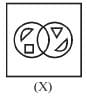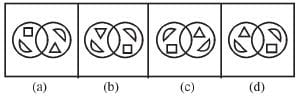Olympiad Test : Mirror Images - 2 - Question 8

In each of the following questions, choose the correct mirror image of the figure (X) from amongst the four alternatives (a), (b), (c) and (d) given along with Fig. (X).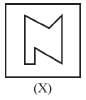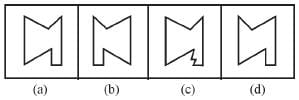Olympiad Test : Mirror Images - 2 - Question 9

In each of the following questions, choose the correct mirror image of the figure (X) from amongst the four alternatives (a), (b), (c) and (d) given along with Fig. (X).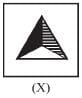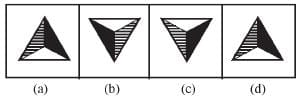Olympiad Test : Mirror Images - 2 - Question 10

In each of the following questions, choose the correct mirror image of the figure (X) from amongst the four alternatives (a), (b), (c) and (d) given along with Fig. (X).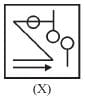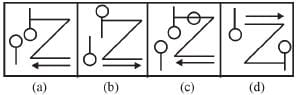## Maths Olympiad Class 6

43 tests
 Use Code STAYHOME200 and get INR 200 additional OFF Use Coupon Code
Information about Olympiad Test : Mirror Images - 2 Page
In this test you can find the Exam questions for Olympiad Test : Mirror Images - 2 solved & explained in the simplest way possible. Besides giving Questions and answers for Olympiad Test : Mirror Images - 2, EduRev gives you an ample number of Online tests for practice

43 tests